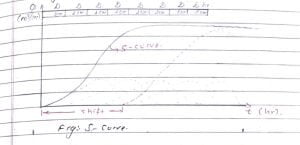Tuesday, October 3, 2023

# Derivation of Unit Hydrograph | Superposition Method & S-Curve Method | Conversion of D-hour to D’ hour unit hydrograph.

### 1. Introduction

Hydrography resulting from one unit depth of excess rainfall (1 cm) generated uniformly over the basin for an effective duration (D hour) is called unit hydrograph.

– Rainfall excess= 1 cm

– Total Depth of rainfall excess = total depth of direct runoff.

– Runoff volume = Δ x 1 cm

Rainfall intensity = 1/D cm/hr

### 2. Assumptions of Unit Hydrograph

a. The excess rainfall has a constant intensity of 1/D cm/hr with an effective storm duration of D hours.

b. Principle of time invariance holds.

c. Unchainging basin characteristics.

d. Uniform distribution of excess rainfall over the basin.

e. Constant base time of DRH for excess rainfall of a given duration.

f. Linear Model: Principle of superposition and proportionality holds.

### 3. Derivation of Unit Hydrograph

For the derivation of unit hydrograph, there are two methods of conversion of D-hour unit hydrograph to D’ hour unit hydrograph.

a. Superposition Method

b. S-Curve Method

a. Superposition Method

If the D-hour unit hydrograph is available, and it is desired to develop a unit hydrograph of nDn, where n is an integer it is accomplished by superposing n unit hydrographs with each graph separated from the previous by D hour. The figure below shows three 4h unit hydrographs A, B, and C.

Curve B begins 4 hr after curve ‘A’ and curves ‘C’ begins 4 hour after ‘B’. Thus, a combination of these curves gives a DRH of 3 cm due to excess rainfall of 12-hour duration. If the ordinate of DRH is now divided by 3, we obtain a 12hr unit hydrograph.b. ‘S’ curve method

If we have to develop a unit hydrograph of m D duration. where m is a fraction, the method of superposition can’t be used.

It is the hydrograph obtained by summation of an infinite series of D-h apart. At any given time the ordinates of the various curves occurring at that time coordinates are summed up to obtain ordinates of s-curve.

The smooth curve through these ordinates results in an S-shaped curve.

The S-hydrograph is a continuously rising curve that ultimately attains a constant value when equilibrium is reached.### 4. Uses of Unit Hydrograph

a. Unit hydrograph can be used to develop the flood hydrographs for extreme rainfall magnitude for the design of the hydraulic structure.

b. It is used for watershed simulation models.

c. It is used for flood forecasting and warning system.

d. Extension of flood flow records based on rainfall records.

e. Once a unit hydrograph is prepared for a duration D hour of a basin, the storm hydrograph for that basin for any other storm of different intensities but the same duration can be developed.

### 5. Limitations of Unit Hydrograph

a. It can’t be applied to very large(A>5000 Km2) and very small (A<2 Km2) catchment areas.

b. Not suitable for the long basin.

c. Applicable for a short duration.

d. Precipitation must be from rainfall only.

e. The UH theory is only ± 10% only.

f. The base period is not exactly the same for all storms.

g. The principle of linearity is not strictly correct.

h. Catchment area shouldn’t have large storage such as tanks, ponds, etc.

i. Not applicable for basins having a high variation of rainfall intensity.He is a founder and lead author of Dream Civil International and his civil engineering research articles has been taken as source by world's top news and educational sites like USA Today, Time, The richest, Wikipedia, etc.
Latest Articles

Related Articles